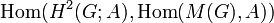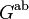# Commutator map in central extension defines homomorphism from Schur multiplier of quotient group to central subgroup

## Statement

### For a fixed choice of extension

Consider a short exact sequence of groups:$0 \to A \to E \to G \to 1$

such that$E$ is a central extension, i.e., the image of$A$ in$E$ is a central subgroup of$E$. Then, this defines a natural homomorphism:$\beta: M(G) \to A$

where$M(G) = H_2(G;\mathbb{Z})$ is the Schur multiplier of$G$.

Another way of thinking of this is that we get a single element$\beta$ of the group$\operatorname{Hom}(M(G),A)$.

Note that$\operatorname{Hom}(M(G),A)$ is a group under pointwise addition because both$M(G)$ and$A$ are abelian groups.

### Varying the extension

Now, suppose we fix an abelian group$A$ and a group$G$ but let$E$ (the central extension group) vary. Then, the possibilities for$E$ up to congruence correspond to the elements of the second cohomology group for trivial group action$H^2(G;A)$. We know that for each extension, we get an element of$\operatorname{Hom}(M(G),A)$. The element is invariant under congruence, i.e., congruent group extensions define the same homomorphism. We thus obtain a set map:$H^2(G;A) \to \operatorname{Hom}(M(G),A)$

This set map is a homomorphism.

Thus, we get an element of$\operatorname{Hom}(H^2(G;A),\operatorname{Hom}(M(G),A))$.

It turns out that this homomorphism is surjective (see the formula for second cohomology group for trivial group action in terms of Schur multiplier and abelianization).

## Extension properties and the relation with the nature of the map

Nature of the map$\beta:M(G) \to A$ Corresponding property of the group extension Explanation
injective the group extension is an initial object in the category of central extensions with homoclinisms for$G$ PLACEHOLDER FOR INFORMATION TO BE FILLED IN: [SHOW MORE]
surjective the group extension is a stem extension PLACEHOLDER FOR INFORMATION TO BE FILLED IN: [SHOW MORE]
bijective the extension group is a Schur covering group PLACEHOLDER FOR INFORMATION TO BE FILLED IN: [SHOW MORE]

## Relation with short exact sequences

### The Stallings exact sequence

Further information: Stallings exact sequence

The first version of the map (#For a fixed choice of extension) is the map$\beta$ in the Stallings exact sequence for a central extension. It can also be used, somewhat indirectly to describe the map$\beta$ for the Stallings exact sequence for a non-central extension.

### The universal coefficients theorem short exact sequence

This short exact sequence relates to the second version #Varying the extension. Explicitly, given an abelian group$A$ and a group$G$, the following short exact sequence exists:$0 \to \operatorname{Ext}^1_{\mathbb{Z}}(G^{\operatorname{ab}},A) \to H^2(G;A) \to \operatorname{Hom}(M(G),A) \to 0$

Note that:

•$G^{\operatorname{ab}}$, the abelianization of$G$, is also the first homology group for trivial group action$H_1(G;\mathbb{Z})$.
•$M(G)$, the Schur multiplier of$G$, is also the second homology group for trivial group action$H_2(G;\mathbb{Z})$.

Thus, the above can be written as:$0 \to \operatorname{Ext}^1_{\mathbb{Z}}(H_1(G;\mathbb{Z}),A) \to H^2(G;A) \to \operatorname{Hom}(H_2(G;\mathbb{Z}),A) \to 0$

Viewed this way, it is a particular case of the dual universal coefficients theorem for group cohomology.

## Explicit description

The map:$H^2(G;A) \to \operatorname{Hom}(M(G),A)$

can be interpreted in terms of the following alternate description of$M(G)$: it is the kernel of the commutator map from$G \wedge G$ to$[G,G]$ (see Schur multiplier is kernel of commutator map homomorphism from exterior square to derived subgroup). Here is how the mapping is defined.

Consider an extension group$E$ corresponding to a particular element of$H^2(G;A)$. Our goal is to use$E$ to explicitly construct a homomorphism from <mathM(G)[/itex] to$A$.

The choice of$E$ determines a commutator mapping$E \wedge E \to [E,E]$. Because$G$ is a quotient of$E$ by a central subgroup, this descends to a commutator mapping$G \wedge G \to [E,E]$ (see commutator map is homomorphism from exterior square to derived subgroup of central extension). Further, we know that composing this with the quotient map$E \to G$ gives the usual commutator mapping$G \wedge G \to [G,G]$.

We are interested in restricting the commutator map$G \wedge G \to [E,E]$ to the kernel of the commutator map$G \wedge G \to [G,G]$. The kernel is the Schur multiplier$M(G)$. Further, the image of this kernel must map to the trivial subgroup when projected to$[G,G]$, hence lies in the kernel of the quotient map from$E$ to$G$, which is$A$. Thus, this restriction is a map from$M(G)$ to$A$. This is the map that we send$E$ to.

### Explicit description using two short exact sequences

The above explicit description can be understood better if we consider the following two short exact sequences. Because the sequences involve possibly non-abelian groups on the quotient side, we denote the trivial group at the right end of these short exact sequences as 1 rather than 0. We have:$0 \to M(G) \to G \wedge G \to [G,G] \to 1$

and$0 \to A \to E \to G \to 1$

Now, the commutator map in$E$ gives a map$G \wedge G \to [E,E]$ because$A$ is central (see commutator map is homomorphism from exterior square to derived subgroup of central extension). Composing with the inclusion of$[E,E]$ in$E$, we get a map$G \wedge G \to E$. We also have the usual inclusion map$[G,G] \to G$. The following diagram commutes:$\begin{array}{ccccccccc} 0 & \to & M(G) & \to & G \wedge G & \to & [G,G] & \to & 1\\ \downarrow && && \downarrow &&\downarrow&& \downarrow\\ 0 & \to & A & \to & E & \to & G & \to & 1\\ \end{array}$

We can construct a unique map$M(G) \to A$ such that the diagram continues to be commutative, and that is the map we seek.$\begin{array}{ccccccccc} 0 & \to & M(G) & \to & G \wedge G & \to & [G,G] & \to & 1\\ \downarrow && \downarrow && \downarrow && \downarrow&& \downarrow\\ 0 & \to & A &\to & E & \to & G & \to & 1\\ \end{array}$

## The homomorphism classifies the extension up to isoclinism

The group$\operatorname{Hom}(M(G),A)$ can be described as the second cohomology group up to isoclinism and the map:$H^2(G;A) \to \operatorname{Hom}(M(G),A)$

takes a group extension and sends it to its equivalence class under isoclinism of group extensions.

To understand why this is so, consider the two short exact sequences above:$\begin{array}{ccccccccc} 0 & \to & M(G) & \to & G \wedge G & \to & [G,G] & \to & 1\\ \downarrow && \downarrow && \downarrow && \downarrow&& \downarrow\\ 0 & \to & A &\to & E & \to & G & \to & 1\\ \end{array}$

Suppose$B$ is the subgroup of$A$ that arises as the image of the homomorphism from$M(G)$. We then have the following two short exact sequences:$\begin{array}{ccccccccc} 0 & \to & M(G) & \to & G \wedge G & \to & [G,G] & \to & 1\\ \downarrow && \downarrow && \downarrow && \downarrow&& \downarrow\\ 0 & \to & B &\to & [E,E] & \to & [G,G] & \to & 1\\ \end{array}$

The right map is the identity map. Now, both the left and right maps are surjective. It is easy to see from this that the middle map is surjective and is determined by the left and right maps. In other words, we have determined from the element of$\operatorname{Hom}(M(G),A)$ the map$G \wedge G \to [E,E]$, even though the congruence type of extension group$E$ is unknown. (Note that this assertion is a special case of the nine lemma). From this, it is clear that the extension has been determined up to isoclinism.